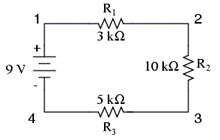Physics
Easy

Question

# Calculate the equivalent resistance of the circuit given below.## 3 kΩ10 kΩ13 kΩ18 kΩHint:

## The correct answer is: 18 kΩ

### The total equivalent resistance in the given circuit is 18 kΩ. The total resistance in a circuit which has a series of resistors can be calculated as the sum of all the resistances. The total resistance in a series Req.= R1+R2+R3 The resistances produced by R1, R2, and R3 are 3kΩ, 10kΩ, and 5kΩ respectively. Hence, R= 3+10+5= 18kΩ is the total equivalent resistance in the given circuit.#### With Turito Foundation.#### Get an Expert Advice From Turito.